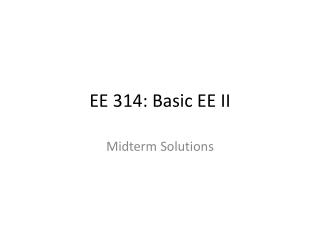DownloadDownload PresentationEE 314: Basic EE II

# EE 314: Basic EE II

Télécharger la présentation## EE 314: Basic EE II

- - - - - - - - - - - - - - - - - - - - - - - - - - - E N D - - - - - - - - - - - - - - - - - - - - - - - - - - -
##### Presentation Transcript

1. EE 314: Basic EE II Midterm Solutions

2. Problem 1 • What makes a material a semiconductor? What are dopants? • A semiconductor acts as an insulator at very low temperatures and as a conductor at very high temperature. It has 4 valence electrons. • Dopants are added to increase the conductivity of a semiconductor. P- type (has 3 valence electrons) and N-type (has 5 valence electrons). • What are N-channel transistors and P-channel transistors? What role does the gate electrode play? • An N-Channel transistor has two heavily doped n+ regions (Source and Drain) separated by a lightly doped p-substrate. A positive gate voltage drives holes in the p-substrate away from the gate and attracts electrons, forming  n-channelunder the gate oxide. • An P-channel transistor is similar but with p+ Source and Drain separated by n-substrate. A negative gate to source voltage is needed to turn on the transistor (form p-channel). • Gatevoltage can lead to the formation of the channel, thus the gate electrode can be used to turn transistor on or off .

3. Problem 1 • Describe how nanotechnology can help to localize cancer cells. • Nano sized gold particles coated with enzymes can be injected into the blood steam. The enzymes are so designed that they only adhere to cancer cells . • These enzymes can either attack and destroy the cancer cells or help in detection and location of the cells. • How does a solar cell works? • A solar cell is similar to an LED. Solar cell excited by light to force electric current whereas an LED uses electric current to emit light. • Light shining on a PN junction provides sufficient energy to electrons in the valence band so that they can move to conduction band, thus generating electricity.

4. Problem 2: Determine the Thevenin equivalent circuit between terminals A and B for the circuit shown in Fig. 2 below. N1 • Consider the open circuit case: - • Applying KCL at node ‘N1’ • [A] • Find the open –circuit voltage VO: - + VO -

5. Problem 2: Contd. + V1 - N2 N1 + • Consider the short circuit case: - • Applying KCL at node ‘N1’ • Find the short –circuit current IO (KCL at node ‘N2’): • Thevenin equivalent resistance: V1 IO -

6. Problem 3: Use load-line analysis to determine ID and VD values for the circuit in Fig. 3(a). The diode characteristic is shown in Fig. 3(b) below. • V = IDRS + VD => 2 = ID*1.5k + VD • Load Line: • If VD = 0, ID = 1.33mA • If ID = 0, VD = 2V • Operating point is intersection of characteristic and load line. • Hence • VD = 0.575V • ID = 0.95mA ID VD

7. Problem 2: Alternative way to find Thevenin resistance. N1 N2 + • Consider the open circuit case: - • Applying KCL at node ‘N2’ • Applying KVL : - Ix Vx -